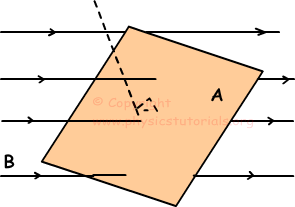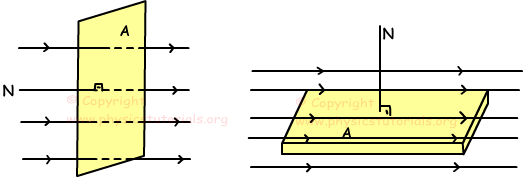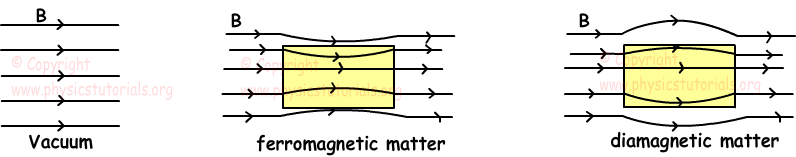التدفق المغناطيسي magnetic flux

### ملخص

Magnetic Flux

Magnetic flux is the number of magnetic field lines passing through a surface placed in a magnetic field.We show magnetic flux with the Greek letter; Ф. We find it with following formula;

Ф=B.A.cosӨ

Where Ф is the magnetic flux and unit of Ф is Weber (Wb)

B is the magnetic field and unit of B is Tesla

A is the area of the surface and unit of A is m2

Following pictures show the two different angle situation of magnetic flux.In the first one, magnetic field lines are perpendicular to the surface, thus, since angle between normal of the surface and magnetic field lines 0 and cos0=1equation of magnetic flux becomes;

Ф=B.A

In the second picture, since the angle between the normal of the system and magnetic field lines is 90º and cos90º=0 equation of magnetic flux become;

Ф=B.A.cos90º=B.A.0=0

Magnetic Permeability

In previous units we have talked about heat conductivity and electric conductivity of matters. In this unit we learn magnetic permeability that is the quantity of ability to conduct magnetic flux. We show it with µ. Magnetic permeability is the distinguishing property of the matter, every matter has specific µ. Picture given below shows the behavior of magnetic field lines in vacuum and in two different matters having different µ.Magnetic permeability of the vacuum is denoted by;µand has value;

µo=4π.10-7Wb./Amps.m

We find the permeability of the matter by following formula;

µ= B / H where; H is the magnetic field strength and B is the flux density

Relative permeability is the ratio of a specific medium permeability to the permeability of vacuum.

µr=µ/µo

Diamagnetic matters: If the relative permeability f the matter is a little bit lower than 1 then we say these matters are diamagnetic.

Paramagnetic matters: If the relative permeability of the matter is a little bit higher than 1 then we say these matters are paramagnetic.

Ferromagnetic matters: If the relative permeability of the matter is higher than 1 with respect to paramagnetic matters then we say these matters are ferromagnetic matters

### فيديو

«الرجوع للقسم»
تصميم وبرمجة المتحدة لخدمات الانترنت

جميع الحقوق محفوظة © لموقع لغة الروح